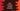# Dart instance variable examples## Introduction :

Instance variables are variables declared inside a class. Every object of that class gets a new copy of instance variables. In this post, we will learn how instance variables work and different ways to initialize them.

## Example of instance variable :

Let’s consider the below example :

``````class Student{
String name;
num age;
}

main() {
var alex = new Student();
alex.name = "Alex";

print("Name : \${alex.name}, Age : \${alex.age}");
}
``````

It will print out :

``````Name : Alex, Age : null
``````

Here, we have two instance variables name and age. After the declaration, both variables have the value null i.e. when the alex object is created, both name and age were initialized with null. Then, we assigned one value Alex to the variable name. So, the print method prints Alex for name variable.

All instance variables have implicit getter method and implicit setter method for all non final variables. Using a dot (.), we can access these variables. You can also use different getter/setter methods to initialize them.

### Initialize instance variables in a constructor :

We can re-write the above program to initialize the instance variables in a constructor when the object

``````class Student {
String name;
num age;

Student(this.name, this.age);
}

main() {
var alex = new Student("Alex", 20);

print("Name : \${alex.name}, Age : \${alex.age}");
}
``````

It will print :

``````Name : Alex, Age : 20
``````

We are initializiing the variable in the constructor and setting their values before the constructor body runs. You can also set the values in the constructor body, but it is concise and easy.

### Initialize instance variables in initializer list :

``````class Student {
String name;
num age;

Student.create(String studentName, num studentAge): name = studentName, age = studentAge {
print("One new student is created with Name : \${this.name} and Age : \${this.age}");
}
}

main() {
var alex = Student.create("Alex", 20);

print("Name : \${alex.name}, Age : \${alex.age}");
}
``````

Initializer list initializes instance variables before the constructor body runs. You can initialize instance variables without using this.

### Initialize final instance variables :

final variables can’t be changed. You can use one normal constructor or constant constructor for that. If you are using constant constructor, all instance variables should be final as these types of objects can’t be changed.

``````class Student {
final String name;
final num age;

const Student(this.name, this.age);
}

main() {
var alex = Student("Alex", 20);

print("Name : \${alex.name}, Age : \${alex.age}");
}
``````

Different ways are there to initialize instance variables. It depends on the program, how to initialize and how to access its values. It is also a good practice to add null check to an instance variable if you are not sure that it got initialized or not.